Electron. J. Differential Equations, Vol. 2021 (2021), No. 14, pp. 1-31.

### Existence and concentration results for fractional Schrodinger-Poisson system via penalization method Zhipeng Yang, Wei Zhang, Fukun Zhao

Abstract: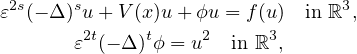where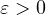is a small parameter,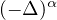denotes the fractional Laplacian of orders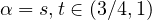,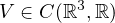is the potential function and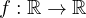is continuous and subcritical. Under a local condition imposed on the potential function, we relate the number of positive solutions with the topology of the set where the potential attains its minimum values. Moreover, we considered some properties of these positive solutions, such as concentration behavior and decay estimate. In the proofs we apply variational methods, penalization techniques and Ljusternik-Schnirelmann theory.Zhipeng Yang Department of Mathematics Yunnan Normal University Kunming 650500, China email: yangzhipeng326@163.com Wei Zhang School of Statistics and Mathematics Yunnan University of Finance and Economics Kunming 650221, China email: weizyn@163.com Fukun Zhao Department of Mathematics Yunnan Normal University Kunming 650500, China email: fukunzhao@163.com